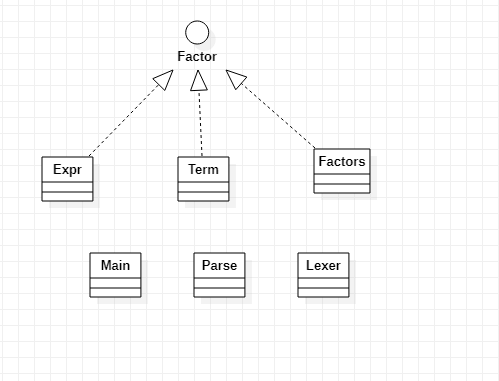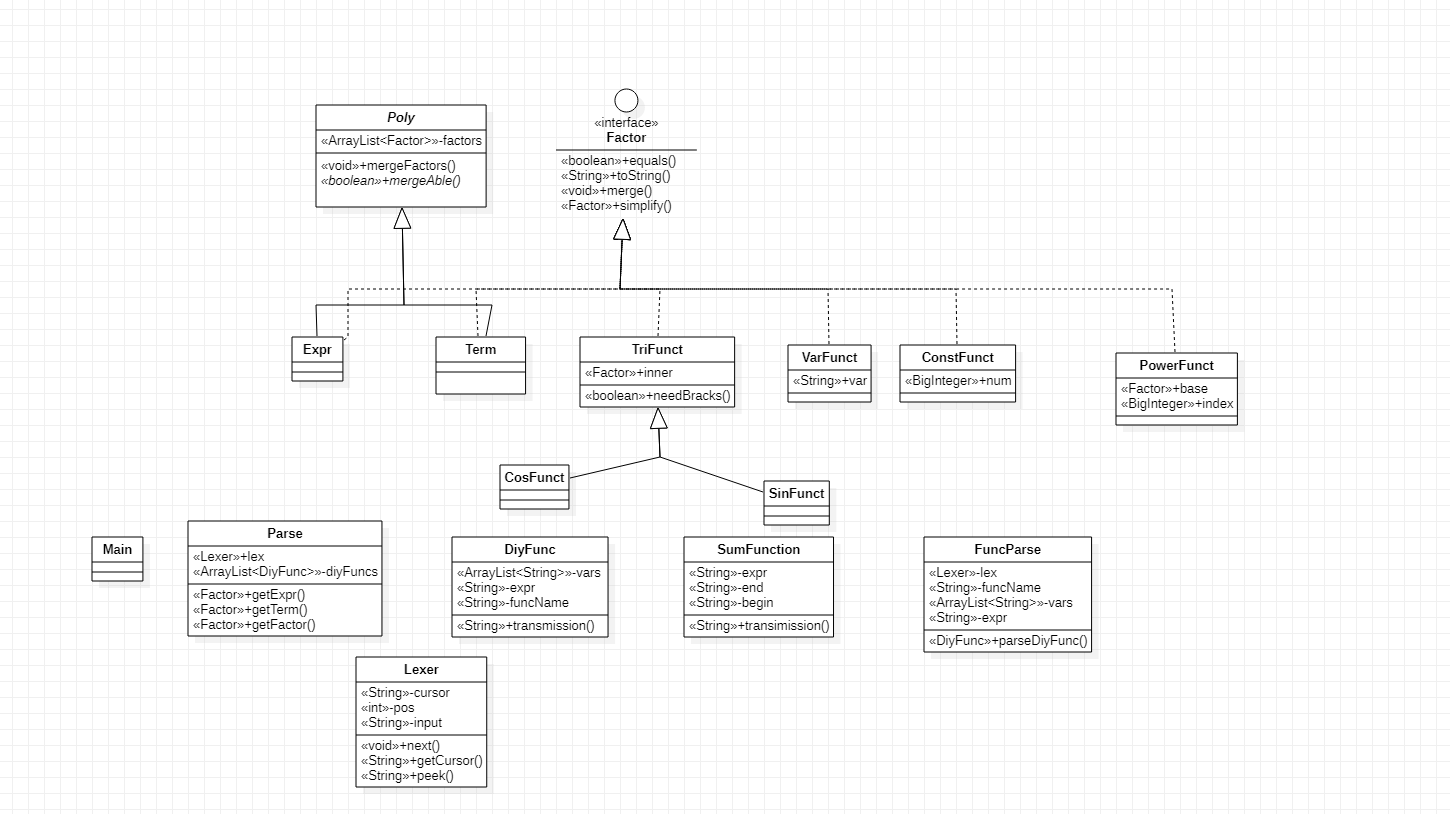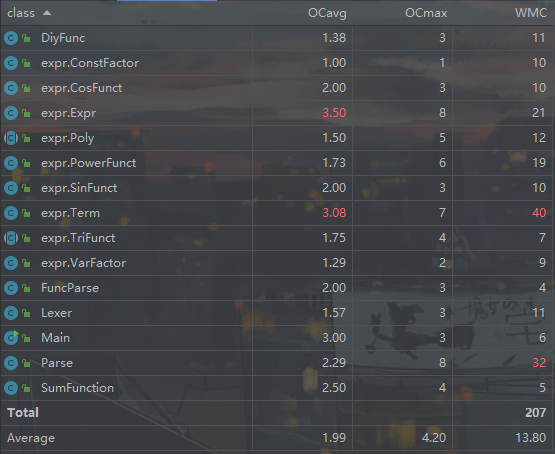# OO_Lab0_表达式的展开

## BNF描述

BNF描述中去除了所有的空白项便于解析

\begin{aligned} 表达式 &\rightarrow[加减] 项| 表达式 加减项\\ 项 &\rightarrow [加减] 因子 | 项'*'因子\\ 因子 &\rightarrow​ 变量因子 | 常数因子 | 表达式因子\\ 变量因子 &\rightarrow 幂函数 | 三角函数 | 自定义函数调用 | 求和函数\\ 常数因子 &\rightarrow​ 带符号的整数\\ 表达式因子 &\rightarrow​ '(' 表达式 ')' [指数]\\ 幂函数 &\rightarrow (函数自变量|'i') [指数]\\ 三角函数 &\rightarrow 'sin''('因子')' [指数] | 'cos''('因子')' [指数]\\ 指数 &\rightarrow '**'['+'] 允许前导零的整数\\ 带符号的整数 &\rightarrow [加减] 允许前导零的整数\\ 允许前导零的整数 &\rightarrow (0|1|2|…|9){0|1|2|…|9}\\ 加减 &\rightarrow '+' | '-' \end{aligned}

## 工作流程

$读入输入 \rightarrow 解析输入 \rightarrow 简化表达式 \rightarrow 输出表达式$

## 抽象结构

### 总体架构

• 表达式类(是一个加法函数,由项类组成)

• 项类(是乘法函数,由表达式组成)

• 因子类

• 变量因子

• 表达式因子

• 常数因子• FuncParse是解析输入的自定义函数定义的类,parseDiyFunc方法返回一个DiyFunc对象.
• DiyFuncSumFunctiontransmission方法是处理输入的自定义函数调用或者求和函数,返回替换之后的字符串.
• PolymargeAble方法是一个抽象方法,留给ExprTerm类自己重写,自定义什么情况两者算同类相,需要合并.
• Factormerge方法是实现接口的类和自己同类型相互合并的方法,该方法不修改对象本身,而是返回一个新的对象.

• 读取函数定义,储存函数的形参,函数名和表达式

• 读取函数调用,获得实参

• 建立实参和形参之间的hashtable

• 遍历表达式,将形参转换成实参

### 细节实现

#### 化简

$C*f_1*f_2 \cdots *f_n$

$(A+B)*(C+D) = A*C+A*D+B*C+B*D$

## 随机数据生成器

• 第一次作业,也是最简单的作业,基于给的BNF还是很好写的,就不赘述了.

• 第二次作业,我原本时在第一次上进行迭代的,但是第二次作业引入了新的自定义函数,自定义函数生成的时候有很多对因子的限制,导致我不得不使用很多变量来限制生成数据时候的选择,比如函数生成时变量的改变,求和函数的求和因子不能时表达式因子等.

• 第三次作业,规则引入更多,而之前的数据生成器已经是300行的屎山代码了,因此我重构代码,引入了DiyFunc和SumFunc两个类来生成自己的数据,做到表达式,自定义函数和求和函数生成的低耦合,再重写__str__方法,直接输出即可.另外对于项的总数和因子总数等也可以定义为全局变量,可以很好的自己控制数据复杂度.


from random import randint

def getExpr(numofTerm = 0):

ret = ""

if(numofTerm == 0):

ret += getTerm()

if(numofTerm< maxTerm and randint(1,100) < 50+numofTerm*10):

ret += getExpr(numofTerm=numofTerm+1)

return ret

def getTerm(numoFactor = 0):

ret = ""

if (numoFactor == 0):

ret += getFactor()

if(numoFactor< maxFactor and randint(1,100) < 50+numoFactor*10):

ret += "*"

ret += getTerm(numoFactor=numoFactor+1)

return ret

def getFactor():

op = randint(0,2)

if(op == 0): ##常数因子

return str(randint(-10,10))

elif(op == 1): ##变量因子

return getVar()

elif(op == 2): ## 表达式因子

ret = "(" + getExpr() + ")"

ret += getIndex()

return ret




import subprocess

sb = subprocess.Popen(command,stdout=subprocess.PIPE,stdin=subprocess.PIPE)

sb.stdin.write(bytes("3\n",encoding = 'utf-8'))

sb.stdin.write(bytes("f(x,y,z)="+F.expr+"\n",encoding='utf-8'))

sb.stdin.write(bytes("g(x,y,z)="+G.expr+"\n",encoding='utf-8'))

sb.stdin.write(bytes("h(x,y,z)="+H.expr+"\n",encoding='utf-8'))

sb.stdin.write(bytes(ExprToJava,encoding = 'utf-8'));

sb.stdin.close();

sb.stdout.close();




if sympify(expr1 - expr2):

print("True")

else :



## debug总结

### 个人bug发现

• 类引用的问题:java对于对象是引用,所以如果两个变量实际是指向同一个对象,那么就会出现神奇的bug.比如(sin(x)**2+cos(x)*sin(x))**2,本来按照乘法分配律展开是

$sin(x)**2*sin(x)**2 + 2 * sin(x)**2*cos(x)*sin(x)+cos(x)*sin(x)*cos(x)*sin(x)$

• -1的解析:在我的架构中-1解析成了-1*1,也就是说输入的每一个带符号整数,在定义中是属于因子,到我的架构里面其实是一个项,但是在后续作业中我忘记了这一个区别,导致抛出了异常.

### 互测bug发现

• 过度优化导致牺牲正确性:后面作业互测中发现了有同学优化了cos(x)**2+sin(x)**2 = 1,这本来是一个很厉害的优化,但是在实际互测中发现有同学的优化是基于原有架构上的拆东墙补西墙,把原本架构的一些细节改变,但没有填补.比如第三次作业,三角函数中可以放表达式因子,那么在判断是否能优化的时候就要判断三角函数里面的因子是否相等,因此有人又修改了表达式因子的equals方法,但这又导致了在项合并同类项中表达式因子合并出现了bug.因此,我认为优化应该是基于能完成需求的基础上,不能为了优化而去优化.

• 放弃优化导致牺牲时间复杂度:我也碰见了有的同学放弃了合并同类项,导致输出巨长,放一个比较复杂的数据,因为没有合并同类项,导致在开括号的时候项一级的因子越来越多,如果指数大一点就直接跑不出结果了.

## 总体总结• 第一次作业我就已经能解决嵌套括号的问题

• 对于新增加的因子我也有了一套比较完整的实现模型

• 对于新增因子的合并我在项和表达式一级也解开了耦合,让这两个类能更好的自己控制合并同类项这一个行为.

posted @ 2022-03-22 20:56  青衫染墨  阅读(380)  评论(1编辑  收藏  举报